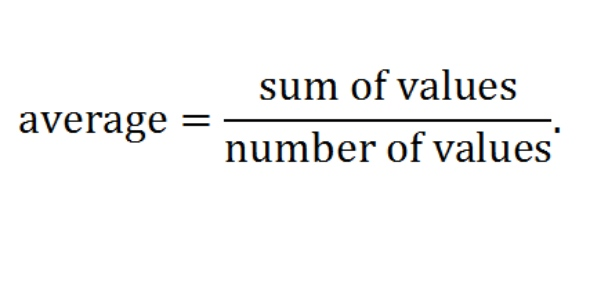What will be your formula for obtaining the average of the following - ProProfs DiscussTopicsMoreProductsMore+ Ask Question

# What will be your formula for obtaining the average of the following cells? A1=90 A2=85 A3=80 A4=75 A5=75Change Image    Delete

This question is part of MICROSOFT EXCEL EXAM
Asked by Wyatt Williams, Last updated: Sep 18, 2020

###Request 0FollowShareAnswer AnonymouslyAnswer LaterCopy LinkA. Cook, English Professor, M.A, Ph.D, Kentucky

The formula to use in obtaining the average of the cells listed above in Microsoft excel is = average (a1:a5).
To get average, you add the values of all the items in the cells divided by the number of cells
= (a1 +a2+ a3 +a4+ a5)/ 5
Therefore, the two possible formulas of obtaining average in Microsoft excel are:
= average (a1:a5).
= (a1 +a2+ a3 +a4+ a5)/ 5
Hope this helps.=AVERAGE(A1:A2),=(A1+A2+A3+A4+A5)/5,=average(a1:a5),=(a1+a2+a3+a4+a5)/5Engmaroof

To get the average of range a1:a5 the formula should be
= average(a1:a5)Londafb

To average, you add the numeric values of all the items and then divide by the NUMBER OFITEMS added.
Capitalization is not an issue...

• =(A1+A2+A3+A4+A5)/5
• =AVERAGE(a1:a5)

I got the question right, but my answer was marked "wrong".Wbonhart

My answer was =average(a1:a5). I did not use uppercase letters. You do not have to use uppercase letters in Excel for the formula to work. So my answer is not wrongJohn Smith=AVERAGE(A1:A5), =(A1+A2+A3+A4+A5)/5, =average(a1:a5), =(a1+a2+a3+a4+a5)/5Search for Google imagesSelect a recommended image
Upload from your computerCancelSearch for Google imagesSelect a recommended image
Upload from your computerCancelSearch for Google imagesSelect a recommended image
Upload from your computerCancel